# C.1 Notation

I shall assume that we have some function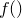, which takes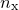parameters,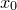...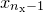, the set of which may collectively be written as the vector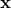. We are supplied a datafile, containing a number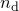of datapoints, each consisting of a set of values for each of theparameters, and one for the value which we are seeking to makematch. I shall call of parameter values for theth datapoint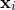, and the corresponding value which we are trying to match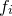. The data file may contain error estimates for the values, which I shall denote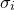. If these are not supplied, then I shall consider these quantities to be unknown, and equal to some constant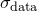.

Finally, I assume that there are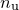coefficients within the functionthat we are able to vary, corresponding to those variable names listed after the via statement in the fit command. I shall call these coefficients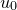...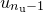, and refer to them collectively as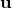.

I model the valuesin the supplied data file as being noisy Gaussian-distributed observations of the true function, and within this framework, seek to find that vector of valueswhich is most probable, given these observations. The probability of any givenis written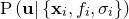.